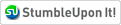# Solar Power Kwh## Calculation of solar energy in your home needs

The calculation of average energy consumption of household

It is estimated that an average U.S. household consumes about 10,000 kWh of energy per year, but every house is different. So let's calculate the energy consumption of your household a year.

For calculate this value, you will need to pool their energy bills over the past 12 months. Look at the values of kWh per month in each account and write each of these values on a sheet of paper. Now add the 12 numbers to obtain total annual value of energy consumption.

As a matter of simple calculations, we assume the annual total power usage is 12,000 kWh (with an average monthly consumption is about 1000 kWh).

Now that you know the total annual electricity needs, it must be combined with a properly sized solar panel. Note that solar panels are rated for peak power and average score, and has to find its average annual production panel.

To put it simply: a 10 kW solar array 12 000 kW / h Estimated annual production, if the panel is installed in a place like Philadelphia (the area has an average of seven hours of sunshine).

Therefore, a panel of 10 kW can be running a house that uses 12 000 kWh of electricity per year in the Philadelphia area. What are the dimensions of a 10 kW system be? Its total area would be about of 700-1000 m. ² The matrix can be composed of 48 small solar panels 210 watts each.

Two important notes:

1. Production estimated at panel may vary widely depending on the geographic location of the house and in the orientation of the panels installed.

2. Retailers often solar panels estimate the average production of a panel to sunny areas of the world as the Mojave Desert in California. It can be estimated a10 kW panel to be as many as 30,000 kW / h per year, which exceeds three times normal value (the value of 30,000 kW / h would be wrong for those living in the Mojave Desert, and wrong that those who live in a temperate zone, such as Philadelphia).

Financial aspects – costs and savings

How much money could a panel of 10 kW saved annually in electrical terms? A grid pattern of the average power tariff in the U.S. is \$ 0.12/kWh. If we multiply 12 000 kWh per U.S. \$ 0.12, we will have a total of \$ 1,440 per year.

A commercial system of this size could cost around \$ 30,000 (after all discounts and credits tributaries, including the tax credit of 30% U.S.). The system would pay for about 20 years and then start generating electricity for free. Building this system your account could save you thousands of dollars by allowing the system to pay for itself much faster.